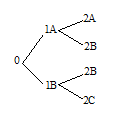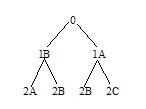### 17.2.3 Examples of CLIM Graph Formatting

`(defstruct node (name "") (children nil))`
` `
`(defvar g1 (let* ((2a (make-node :name "2A"))`
`                  (2b (make-node :name "2B"))`
`                  (2c (make-node :name "2C"))`
`                  (1a (make-node :name "1A" :children (list 2a 2b)))`
`                  (1b (make-node :name "1B" :children (list 2b 2c)))) `
`             (make-node :name "0" :children (list 1a 1b))))`
` `
`(defun test-graph (root-node &rest keys) `
`  (apply #'clim:format-graph-from-root root-node `
`         #'(lambda (node s)`
`             (write-string (node-name node) s)) `
`         #'node-children keys)) `

Evaluating ``` (test-graph g1 :stream *my-window*)``` results in the following graph:###### Figure 32. A Horizontal Graph

In Figure 32., the graph has a horizontal orientation and grows toward the right by default. We can supply the :orientation keyword to control this. Evaluating ``` (test-graph g1 :stream *my-window* :orientation :vertical)``` results in the following graph:###### Figure 33. A Vertical Graph

The following example uses format-graph-from-roots to create a graph with multiple parents, that is, a graph in which node D is a child of both nodes B and C. Note that it interprets its first argument as a list of top-level graph nodes, so we have wrapped the root node inside a list.

`(defun test-graph (win)`
`  (window-clear win)`
`  (format-graph-from-roots '((a (b (d)) (c (d))))`
`                           #'(lambda (x s) (princ (car x) s))`
`                           #'cdr`
`                           :stream win`
`                           :orientation :vertical`
`                           :merge-duplicates t`
`                           :duplicate-key #'car)`
`  (force-output win))`

Common Lisp Interface Manager 2.0 User's Guide - 7 Aug 2017